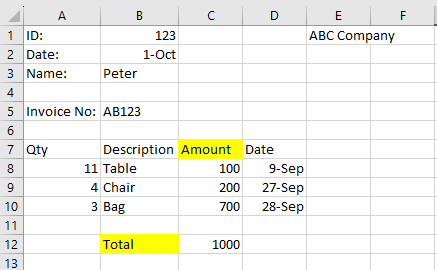# Find Row Index and Column Index by cell value only

How to find row index and column index be cell value only?
I have no column name, column number, or anything else except cell value.

I want to get the row index of “Total” by using string “Total”, expected result is 12
and get the column of “Amount” by using string “Amount”, expected result is “C”

Below is the sample of the excel files, it is dynamic that “Amount” might be in column D, or E, etc in other excelBlankProcess12.zip (53.4 KB)

Hope this helps!!

1 Like

Thanks, didn’t realized there is an activity for this lol

Is there any way to do it without “Use Excel File”?
E.g. read range workbook as DT, and perform linq

Prepare an empty datatatable with build datatable and configure the following columns:
search (string) ,ridx (int32), cidx (int32) - dtReport

Prepare a variable
arrSearchKeys | String Array = new String(){“AMOUNT”, “TOTAL”}

Assign Activity
dtReport =

``````(From c in Enumerable.Range(0, YourDataTableVar.Columns.Count)
From  s in arrSearchKeys
Let i = YourDataTableVar.AsEnumerable.ToList.FindIndex(Function (d) d(c).Tostring.ToUpper.Trim.Equals(s))
Where i > -1
Let ra = new Object(){s, i, c}
``````
1 Like

Hi @mlellison ,

Could you maybe check the below workflow :
DT_GetRowColumnIndices_BasedOnKeywords.zip (9.2 KB)

`Read Range` activity and get Output as Datatable without `Add Headers` enabled is assumed to be done already. Let the datatable variable be named as `DT`.

Using Linq, we could maybe try with the below :

``````DT.rows.IndexOf(DT.AsEnumerable.Where(Function(x)x.ItemArray.Any(Function(y)y.ToString.ToLower.Equals("total"))).First)+1
``````

For Getting the Column Letter we can first find the column Index like below :

``````amountColIndex = DT.AsEnumerable.Select(Function(x)Array.IndexOf(x.ItemArray.Select(Function(y)y.ToString).ToArray,"Amount")).Where(Function(x)x<>-1).FirstOrDefault
``````

We can then use the below Expression to get the Column Letter :

``````amountColExcelLetter = UiPath.Excel.Helpers.ExcelUtilities.ConvertColumnIndexToColumnLetter(amountColIndex+1)
``````